# Part 7 - Parameter Studies¶

## 7.1 The goal¶

We want to run the simulation with a different number of nodes, and see how the behavior of the network changes. With OMNeT++ you can do parameter studies, which are multiple simulation runs with different parameter values.

We'll make the number of central nodes (the "handle" in the dumbbell shape) a parameter, and use the same random routing protocol as before. We're interested in how the average hop count depends on the number of nodes.

## 7.2 Making the network topology parametric¶

To parameterize the network, the number of nodes is given as a NED parameter, `numCentralNodes`. This parameter specifies how many nodes are in the central section of the network, but doesn't cover the two nodes at each side.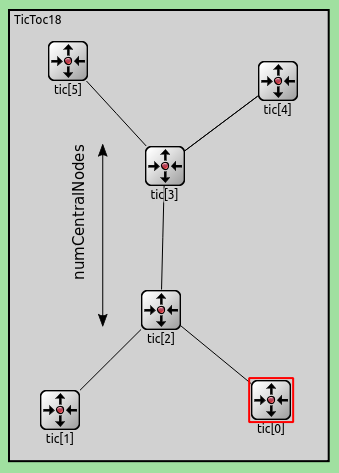The total number of nodes including the four nodes on the sides is `numCentralNodes+4`. The default of the `numCentralNodes` parameter is 2, this corresponds to the network in the previous step.

Now, we must specify that the variable number of nodes should be connected into the dumbbell shape. First, the two nodes on one side is connected to the third one. Then the the last two nodes on the other side is connected to the third last. The nodes in the center of the dumbbell can be connected with a for loop. Starting from the third, each *i*th node is connected to the *i+1*th.

Here is how the network looks like with `numCentralNodes = 4`: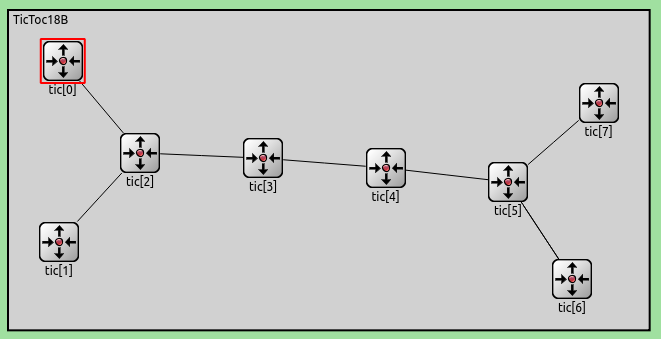To run the simulation with multiple different values of `numCentralNodes`, we specify the variable N in the ini file:

## 7.3 Setting up a parameter study¶

We specify that N should go from 2 to 100, in steps of 2. This produces about 50 simulation runs. Each can be explored in the graphical user interface, but simulation batches are often run from the command line interface using the Cmdenv runtime environment.

Tip

You can find more information on variables and parameter studies in the Parameter Studies section of the OMNeT++ manual.

To increase the accuracy of the simulation we may need to run the same simulation several times using different random numbers. These runs are called Repetitions and are specified in `omnetpp.ini`:

This means that each simulation run will be executed four times, each time with a different seed for the RNGs. This produces more samples, which can be averaged. With more repetitions, the results will increasingly converge to the expected values.

## 7.4 Running the parameter study¶

Now, we can run the simulations. In the dropdown menu of the Run icon, select Run Configurations.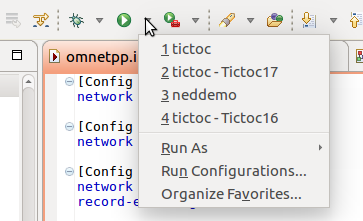In the Run Configurations dialog, select the config name, make sure Cmdenv is selected as the user interface.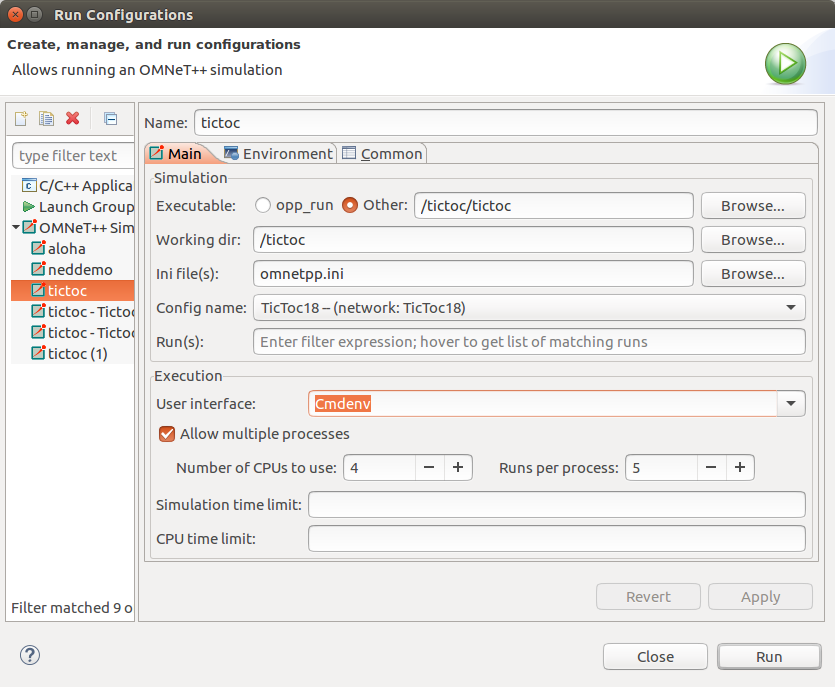If you have a multicore CPU, you can specify how many simulations to run concurrently.

Note

Alternatively, you can run the simulation batches from the command line with `opp_runall` tool with the following command:

```opp_runall -j4 ./tictoc -u Cmdenv -c TicToc18
```

The `-j` parameter specifies the number of CPU cores, the `-u` parameter the user interface, and `-c` the config to run.

## 7.5 Analyzing the results¶

Now, we can visualize and analyze the data we've collected from the simulation runs. We'll display the average hop count for messages that reach their destinations vs N, the number of central nodes. Additionally, we will display the average number of packets that reached their destinations vs N. The analysis file `Tictoc18.anf` contains the dataset we will use for the visualization.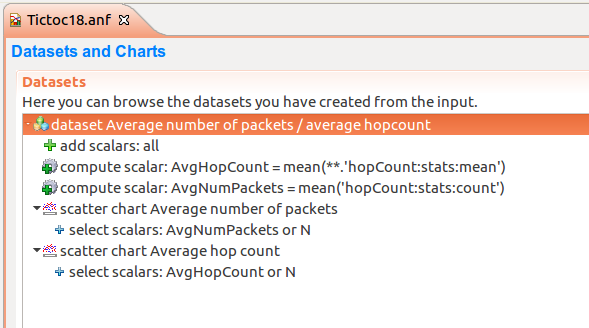These two average scalars are not recorded during the simulation, we will have to compute them from the available data.

The hop count is recorded at each node when a message arrives, so the mean of hop count will be available as a statistic. But this is recorded per node, and we're interested in the average of the mean hop count for all nodes. The 'Compute Scalar' dataset node can be used to compute scalar statistics from other scalars. We compute `AvgHopCount` as `mean(**.'hopCount:stats:mean')`.

We're also interested in the average number of packets that arrive at their destination. The count of the arrived packets is available at each node. We can compute their average, `AvgNumPackets` as `mean('hopCount:stats:count')`.

Tip

Refer to the chapter "Using the Analysis Editor" in the User Guide for more information on datasets. You can find it in the `doc/` directory of your OMNeT++ installation.

Then, we plot these two computed scalars against N in two scatter charts. The data for different repetitions is automatically averaged. Here is the average hop count vs N: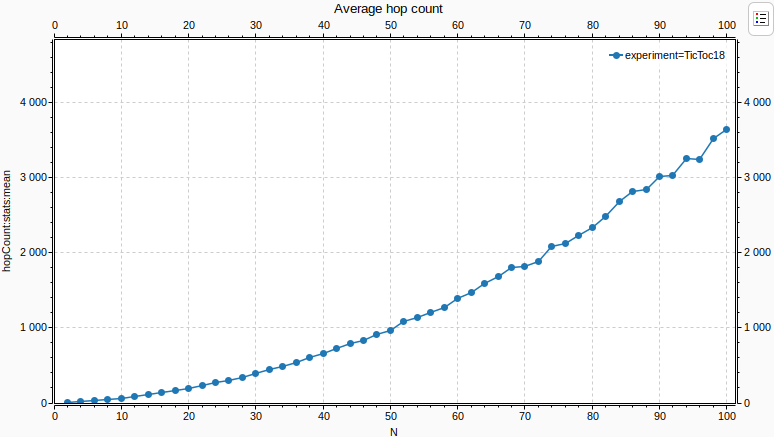The average hop count increases as the network gets larger, as packets travel more to reach their destination. The increase is polynomial. Notice that there are missing values at the far right of the chart. This is because in such a large network, some packets might not reach their destination in the simulation time limit. When no packets arrive at a node, the hop count statistic will be NaN (not a number) for that node. When there is a NaN in any mathematical expression, its result will be also NaN. Thus it takes just one node in all the simulation runs to have a NaN statistic, and the average will be NaN, and there'll be no data to display. This can be remedied by increasing the simulation time limit, so more packets have a chance to arrive.

Below is the average number of packets that arrived vs N: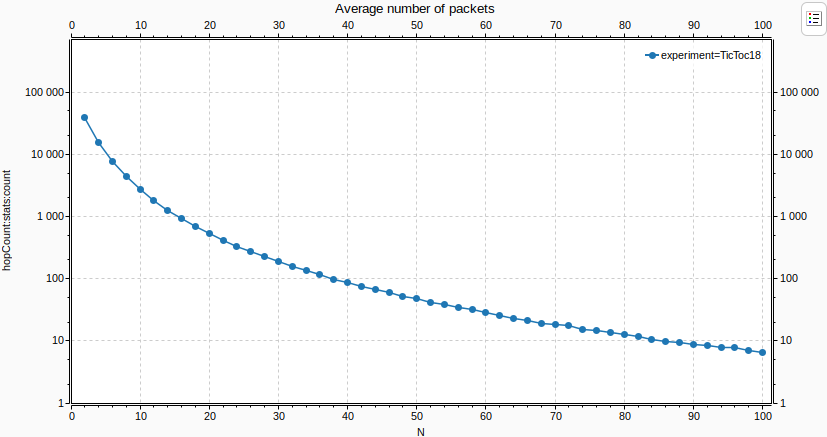Notice that the Y axis is logarithmic. The average number of packets that arrive decreases polynomially as N increases, and the network gets larger.